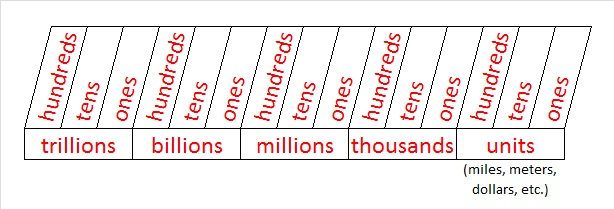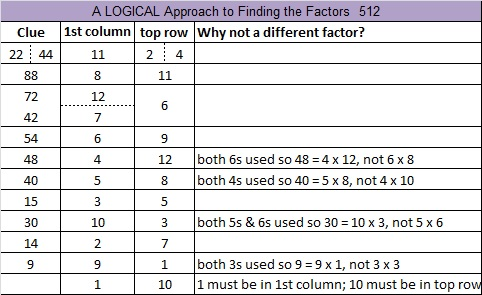# 578 When I stopped teaching about thousands, it made a BIG difference

Several weeks ago I helped a young girl with a place value page in her workbook. The largest number she needed to write out in words was less than 900 million.

The young girl was very confused so I explained it exactly the same way the book explained it – which was exactly the same way it was explained to me when I was a kid.

She easily understood the ones place, the tens place, the hundreds place, and the thousands place.  The trouble began when we started working with the ten thousands place and became even worse when I started talking about the hundred thousands place, the millions place, the ten millions place, and the hundred millions place.

The young girl could easily and correctly separate the digits of any multi-digit number into groups of three, but reading or writing that number using words baffled her.

Also translating number words back into digits and putting those digits into the right places was equally challenging for her.

After explaining what to do on EVERY single problem, I knew she really didn’t get it….even though the assignment was finally finished.

Then last week on twitter I saw a retweet of this:

I wondered what crazy, radical thing was meant by that statement so I clicked on the link and read a very clear and easy-to-understand explanation of how to teach place value. I was very impressed.

This last Friday I saw that young girl again, and I said, “I want to show you something.”

I took my red pen and wrote in her workbook,and this time I actually taught her the concept of place value. This time she got it!

Triumphantly she wrote down a 14-digit number made from some “random” numbers that popped into her head and then read it to me perfectly. It was as thrilling for me as it was for her!

An hour before she couldn’t correctly read 90% of the whole numbers less than a million, but now she had MASTERED thousands, millions, billions, and even trillions.

I really like that blogger/tweeter Michael Tidd chose “units” as the last category because that is the natural place to say miles, meters, dollars or whatever the unit happens to be. The unit this young girl chose for her 14-digit number was CATS.

———————————————————————–

578 is the hypotenuse of two Pythagorean triples: 322-480-578 and 272-510-578. Each triple has a greatest common factor. Which factors of 578 could they be?

• 578 is a composite number.
• Prime factorization: 578 = 2 x 17 x 17, which can be written 578 = 2 x (17^2)
• The exponents in the prime factorization are 1 and 2. Adding one to each and multiplying we get (1 + 1)(2 + 1) = 2 x 3  = 6. Therefore 578 has exactly 6 factors.
• Factors of 578: 1, 2, 17, 34, 289, 578
• Factor pairs: 578 = 1 x 578, 2 x 289, or 17 x 34
• Taking the factor pair with the largest square number factor, we get √578 = (√289)(√2) = 17√2 ≈ 24.04163# 512 Ants and Level 4The 512 Ants on Sullivan Street is an entertaining book for children that also includes learning activities by Marilyn Burns. The book teaches the important concept of doubling in a very natural way, but it can teach even more than that.  Here is a place value lesson for third grade students that uses the same book. There are relationships between adding, subtracting, doubling, multiplying, dividing, powers, and place value. The book and the place value lesson will make some of those relationships clear.

512 is a perfect cube because 512 = 8^3. It is also a cube of a cube because 8 = 2^3. Thus 512 = (2^3)^3 = 2^9. All of the factors of 512 are powers of 2.Print the puzzles or type the solution on this excel file: 12 Factors 2015-06-01

—————————————————————————————————

• 512 is a composite number.
• Prime factorization: 512 = 2 x 2 x 2 x 2 x 2 x 2 x 2 x 2 x 2, which can be written 512 = (2^9)
• The exponent in the prime factorization is 9. Adding one we get (9 + 1) = 10. Therefore 512 has exactly 10 factors.
• Factors of 512: 1, 2, 4, 8, 16, 32, 64, 128, 256, 512
• Factor pairs: 512 = 1 x 512, 2 x 256, 4 x 128, 8 x 64, or 16 x 32
• Taking the factor pair with the largest square number factor, we get √512 = (√256)(√2) = 16√2 ≈ 22.627417—————————————————————————————————